Include Top

# Mood's Median Test - Exact

This tool is used to compute exact P-Values. Typically this would not be necessary unless the sample sizes were smaller (each sample N <= 10 for Mood’s Median), but this gives continuity on the example. Unlike Kruskal-Wallis, computing an exact P-Value is efficient due to the reduction of the data to a Chi-Square table and the use of a Network Model (see Appendix Exact and Monte Carlo P-Values for Nonparametric and Contingency Tests)

1. Open Customer Data.xlsx, click on Sheet 1 tab (or press F4 to activate last worksheet).
2. Click SigmaXL > Statistical Tools > Nonparametric Tests - Exact> Mood’s Median Test - Exact. If necessary, check Use Entire Data Table. click Next.
3. With Stacked Column Format checked, select Overall Satisfaction, click Numeric Data Variable (Y) >>; select Customer Type, click Group Category (X) >>. Select Exact with the default Time Limit for Exact Computation = 60 seconds. Set Values Equal to Overall Median: to Counted as Below.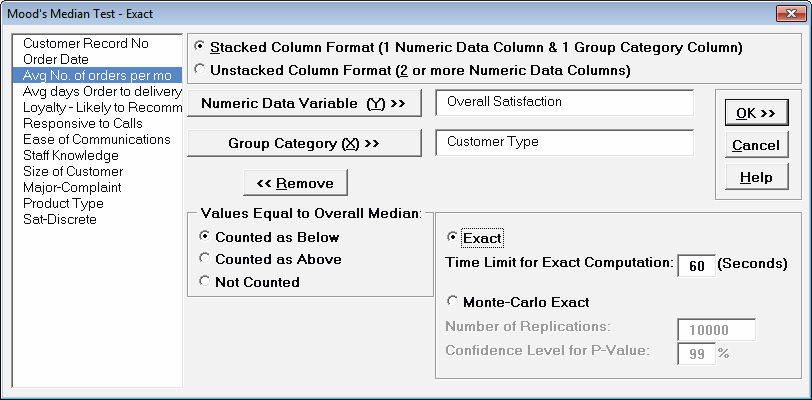Tip
: If none of the observations are equal to the overall median, the options for “Values Equal to Overall Median:” do not affect the exact P-Value. That is the case with this Customer Data example. In cases where there are observations equal to the overall median, then the exact P-Value will be different for each option selected. For regular Mood’s Median, SigmaXL uses Counted as Below (in agreement with Minitab) but will use Counted as Above for small sample cases if the average cell expected value is higher. StatXact uses Counted as Below for Mood’s Median. Matlab uses Not Counted. Users should try Counted as Below and Counted as Above to ensure that the P-Values agree on reject or fail to reject H0. Use of this option will be demonstrated in the small sample example.

Tip: If the exact computation time limit is exceeded a dialog will prompt you to use Monte Carlo or to increase the computation time. When this occurs, Monte Carlo is recommended.

4. Click OK. Resulting Output:Click on cell B17 to view the Exact P-Value with more decimal place precision Given the P-Value of .00124 we reject H0 and conclude that at least one pairwise set of medians are not equal. The Exact P-Value is very close to the above “large sample” or “asymptotic” result (.00121). This was expected because the sample size is reasonable (N > 10), so the “large sample” Mood’s Median P-Values are valid using a chi-square approximation. Note also that the counts in B8:D9 form a two way contingency table and the expected counts are all greater than 5.

The Exact P-Value was computed in seconds, but if the data set was larger, the required computation time could become excessive, and Monte Carlo would be required.

5. Press F3 or click Recall SigmaXL Dialog to recall last dialog. Select Monte Carlo Exact with the Number of Replications = 1e6 and Confidence Level for P-Value = 99%. One million replications are used because the expected P-Value is small as estimated from the “large sample” Mood’s Median above. This will take up to a minute to run, so if you have a slow computer, use 1e5 replications instead of 1e6.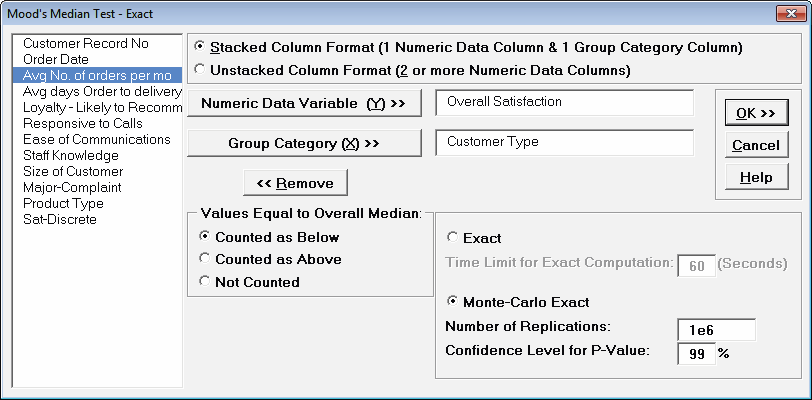Tip: The Monte Carlo 99% confidence interval for P-Value is not the same as a confidence interval on the test statistic due to data sampling error. The confidence level for the hypothesis test statistic is still 95%, so all reported P-Values less than .05 will be highlighted in red to indicate significance. The 99% Monte Carlo P-Value confidence interval is due to the uncertainty in Monte Carlo sampling, and it becomes smaller as the number of replications increases (irrespective of the data sample size). The Exact P-Value will lie within the stated Monte Carlo confidence interval 99% of the time.

6. Click OK.Click on cell B17 to view the Monte Carlo P-Value with more decimal place precision. The Monte Carlo P-Value here is .001249 with a 99% confidence interval of .00117 (B19) to .00133 (B18). This will be slightly different every time it is run (the Monte Carlo seed value is derived from the system clock). The true Exact P-Value = .00124 lies within this confidence interval.

So we reject H0: at least one pairwise set of medians are not equal.

7. Now we will consider the small sample problem used in Kruskal-Wallis. Open Snore Study.xlsx. This data is from: Gibbons, J.D. and Chakraborti, S. (2010). Nonparametric Statistical Inference (5th Edition). New York: Chapman & Hall, (Example 10.2.1, pp. 347-348).

An experiment was conducted to determine which device is the most effective in stopping snoring or at least in reducing it. Fifteen men who are habitual snorers were divided randomly into three groups to test the devices. Each man’s sleep was monitored for one night by a machine that measures amount of snoring on a 100-point scale while using a device.

8. Select Snore Study Data tab. Click SigmaXL > Statistical Tools > Nonparametric Tests – Exact > Mood’s Median Test - Exact. If necessary, check Use Entire Data Table, click Next.
9. With Unstacked Column Format checked, select Device A, Device B and Device C, click Numeric Data Variables (Y) >>. Select Exact with the default Time Limit for Exact Computation = 60 seconds.10. Click OK. Results: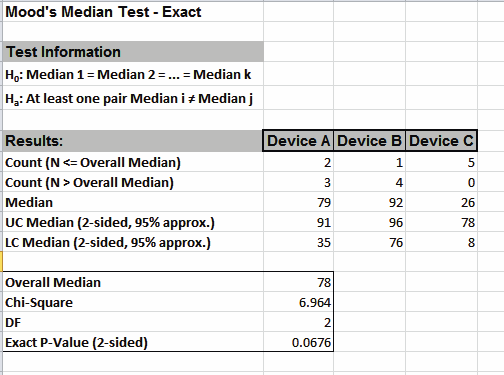With the Exact P-Value = .0676 we fail to reject H0, and cannot conclude that there is a significant difference in median snore study scores. This exact P-Value matches that given in the reference textbook using StatXact. Comparing Mood’s Median to Kruskal-Wallis we see that the Exact P-Value is higher because Mood’s Median is not as powerful as Kruskal-Wallis.

We will now rerun the analysis using the Counted as Above option.

11. Press F3 or click Recall SigmaXL Dialog to recall last dialog. Set Values Equal to Overall Median: to Counted as Above.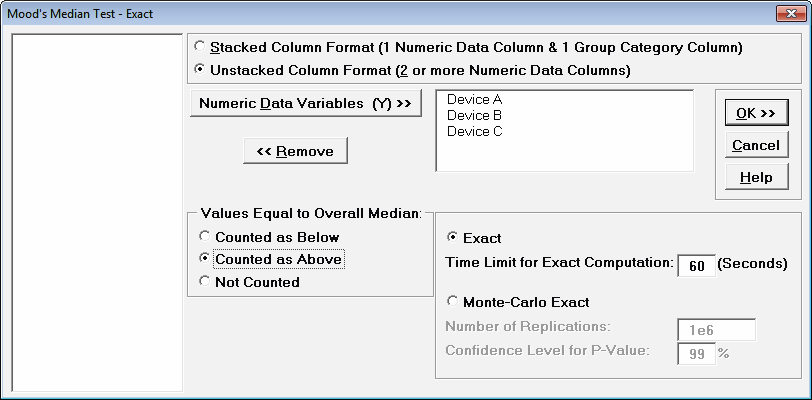12. Click OK. Results: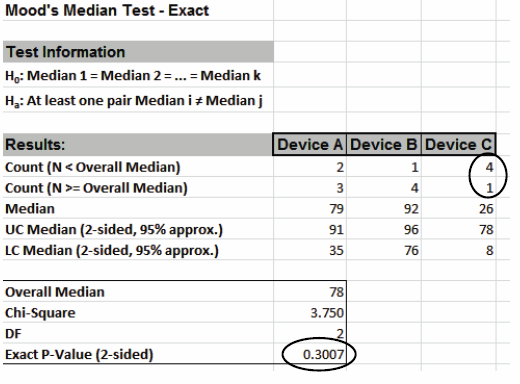With the Exact P-Value = 0.3007 we fail to reject H0, and cannot conclude that there is a significant difference in median snore study scores. Note that setting Values Equal to Overall Median to Counted as Above resulted in a different count for Device C, which results in the dramatic difference in Exact P-Value.

In conclusion, when using Mood’s Median Exact, always try Counted as Below and Counted as Above to ensure that the P-Values agree with each other.

# Web Demos

Our CTO and Co-Founder, John Noguera, regularly hosts free Web Demos featuring SigmaXL and DiscoverSim